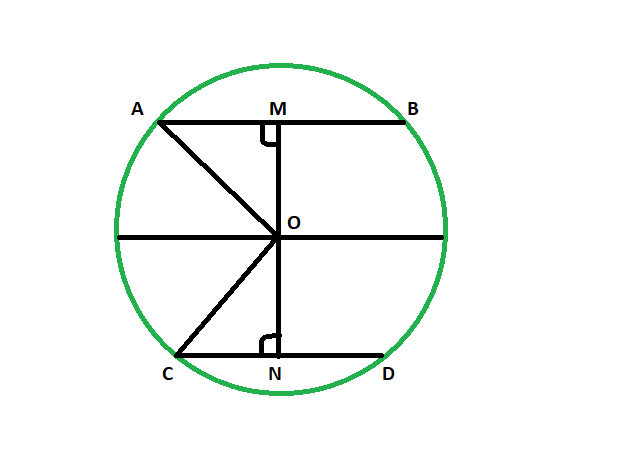# Distance of chord from center when distance between center and another equal length chord is given

Given two equal length chords of a circle and Distance between the centre and one chord. The task is here to find the distance between the centre and the other chord.

Examples:

```Input: 48
Output: 48

Input: 82
Output: 82

```Below is the implementation of the above approach:

Approach:
Let AB & CD be the two equal chords of the circle having center at O.OM be the given distance of the chord AB from center.
now in triangle AOM and CON,
OA = OC (radii of same circle)
MA = CN (since OM and ON are the perpendicular to the chord and it bisects the chord and AM = MB & CN = CD)
angle AMO = angle ONC = 90 degrees
so the triangles are congruent
so, OM = ON

Equal chords of a circle are equidistant from the centre of a circle.

Below is the implementation of the above approach:

## C++

 `// C++ program to find the distance of chord ` `// from center when distance between center ` `// and another equal length chord is given ` ` `  `#include ` `using` `namespace` `std; ` ` `  `void` `lengequichord(``int` `z) ` `{ ` `    ``cout << ``"The distance between the "` `         ``<< ``"chord and the center is "` `         ``<< z << endl; ` `} ` ` `  `// Driver code ` `int` `main() ` `{ ` `    ``int` `z = 48; ` `    ``lengequichord(z); ` `    ``return` `0; ` `} `

## Java

 `// Java program to find the distance of chord ` `// from center when distance between center ` `// and another equal length chord is given/ ` ` `  `import` `java.io.*; ` ` `  `class` `GFG ` `{ ` `     `  `static` `void` `lengequichord(``int` `z) ` `{ ` `    ``System.out.println (``"The distance between the "``+ ` `    ``"chord and the center is "``+ z ); ` `} ` ` `  `// Driver code ` `public` `static` `void` `main (String[] args)  ` `{ ` ` `  `    ``int` `z = ``48``; ` `    ``lengequichord(z); ` `} ` `} ` ` `  `// This code is contributed by jit_t. `

## Python 3

 `# Python 3 program to find the distance of chord ` `# from center when distance between center ` `# and another equal length chord is given ` ` `  `def` `lengequichord(z): ` `    ``print``(``"The distance between the"` `,  ` `          ``"chord and the center is"` `, z ) ` ` `  `# Driver code ` `if` `__name__ ``=``=` `"__main__"``: ` `    ``z ``=` `48` `    ``lengequichord(z) ` ` `  `# This code is contributed  ` `# by ChitraNayal `

## C#

 `// C# program to find the distance of chord  ` `// from center when distance between center  ` `// and another equal length chord is given ` `using` `System; ` ` `  `class` `GFG  ` `{  ` `     `  `    ``static` `void` `lengequichord(``int` `z)  ` `    ``{  ` `        ``Console.WriteLine(``"The distance between the "``+  ` `        ``"chord and the center is "``+ z );  ` `    ``}  ` `     `  `    ``// Driver code  ` `    ``public` `static` `void` `Main ()  ` `    ``{  ` `     `  `        ``int` `z = 48;  ` `        ``lengequichord(z);  ` `    ``}  ` `}  ` ` `  `// This code is contributed by AnkitRai01  `

Output:

```The distance between the chord and the center is 48
```

Attention reader! Don’t stop learning now. Get hold of all the important DSA concepts with the DSA Self Paced Course at a student-friendly price and become industry ready.

My Personal Notes arrow_drop_upCheck out this Author's contributed articles.

If you like GeeksforGeeks and would like to contribute, you can also write an article using contribute.geeksforgeeks.org or mail your article to contribute@geeksforgeeks.org. See your article appearing on the GeeksforGeeks main page and help other Geeks.

Please Improve this article if you find anything incorrect by clicking on the "Improve Article" button below.

Improved By : jit_t, AnkitRai01, chitranayal

Article Tags :
Practice Tags :

Be the First to upvote.

Please write to us at contribute@geeksforgeeks.org to report any issue with the above content.